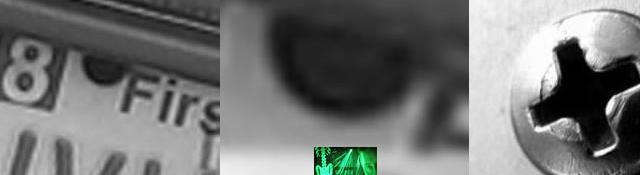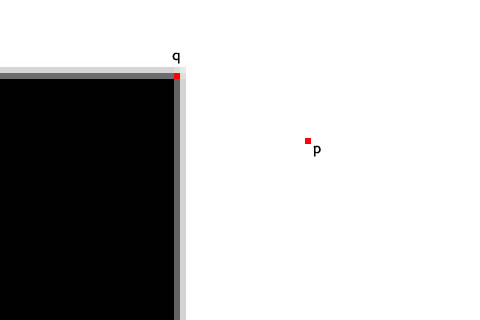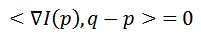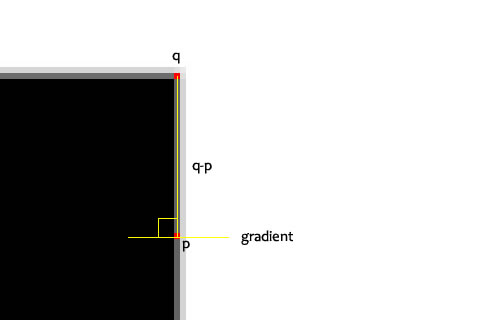## Fundamentals of Features and Corners

A lot of computer vision algorithms use features as their backbone. But what exactly is a feature?

## Subpixel Corners: Increasing accuracy

Series: Fundamentals of Features and Corners:

1. Features: What are they?
2. Harris Corner Detector
3. Interesting windows in the Harris Corner Detector
4. The Shi-Tomasi Corner Detector
5. Subpixel Corners: Increasing accuracy

When working with images on a digital system, the smallest part of an image is a pixel. You simply cannot access information "between" pixels. But several application require higher accuracy than a camera can provide. For example, when reconstructing a 3D object from an image, you need accurate measurements. So, mathematical techniques were developed to increase the accuracy of detecting corners. Oh, and the infinite zoom that you see on CSI: Miami, we won't be doing that.

## Why subpixel accuracy?

Here's a picture of a square. I've zoomed in a lot, so you can see individual pixels. Try finding the corner in this image.You can see that the corner does not lie on a single pixel. The corner is "spread out" (In fact, in real life situations, it's almost impossible to get corners to lie on exact pixels).

So, with a corner detection algorithm like the Shi-Tomasi corner detector or the Harris corner detector, you will end up with a corner like (56, 120). But, scientists and other people want a corner like (56.768, 120.1432).

This is subpixel accuracy. You cannot access pixel (56.768, 120.1432), yet you have determined that the corner lies precisely at this point.

But, why go into the trouble of figuring out the decimal portion? Corners extremely important features in an image. And you need the extra precision for many situations:

• Tracking
• Camera calibration
• 3D reconstruction
• Stereo vision

So a lot of effort has been put into getting better accuracy, faster.

## The technique

We'll go over a basic technique. It uses the familiar dot product (yes, the one you learned in school). A little bit of review about the dot product.

The dot product <a, b> is zero if one of the following holds (a and b are vectors):

1. Either a or b is zero
2. Vectors a and b are perpendicular to each other (then cos90o = 0)

Simple enough? Great! With that in mind, we dive into the algorithm.

The aim of the algorithm is to create several equations. On solving these equations, you increase the accuracy of the corner. Now, how do you create these equations? It's a little trick that is employed.

You take two points, p and q on the image. q is the estimated corner position (the one with integer coordinates). p is any point around q.

It would look something like this:Now, the point q lies very near to the corner. So, p can lie at only one of the following two places:

1. A flat region
2. On an edge

In the above example, if you move p around, you'll see why.

Lets consider option 1 first: a flat region

## Flat regions

Assume p lies on a flat region. This means the gradient at p is zero (the rate of change is zero). So, the following dot product will always be zero (whatever the position of q):The dot product of the two vectors (the gradient at p, and the vector q-p) will be zero. This is because the gradient is always zero.

## On edges

When p lies on an edge, the gradient will be some definite vector (because the pixel values are changing). Also, q-p will be a vector. But in this case, the two will be perpendicular. Here's an illustration:So again, the dot product will be zero:This time, the two vectors are perpendicular to each other (or, orthogonal). So the dot product is zero.

## Several equations

Using several such equations, you can form a system of equations. Each equation equal to zero. And when you solve for q, you get a higher accuracy corner.

Its as simple as that.

## Summary

The key idea in generating several equations. To do this, the dot product is used. Using the approximate corner point and several points around it, many equations are formed. All these equations equal to zero. And on solving for the corner, you get a point with higher precision. It has digits after the decimal.

There also exist other techniques to get subpixel corners. Like iterative algorithms that try to minimize error, etc. But we won't go into those right now.

OpenCV uses this technique to refine corner positions calculated by other techniques (like the Shi-Tomasi corner detector or the Harris corner detector).

## More in the series

This tutorial is part of a series called Fundamentals of Features and Corners:

1. Features: What are they?
2. Harris Corner Detector
3. Interesting windows in the Harris Corner Detector
4. The Shi-Tomasi Corner Detector
5. Subpixel Corners: Increasing accuracy

#### Related posts#### 7 unique neural network architectures